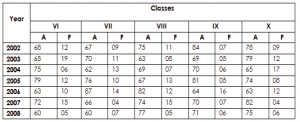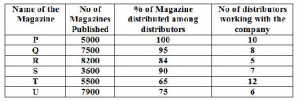## Data Interpretation For SBI PO : Set – 40

D.1-5) Study the table carefully to answer the questions that follow:

Number of Students Appeared (A) and Failed (F) in Five Classes of a School over the Years1) What is the total number of failed students from class VIII for the given years?

a) 77

b) 83

c) 68

d) 71

e) None of these

d)

Total number of failed students from class VIII for the given years =

11+8+7+13+12+15+5 = 71

2) What is the ratio of the total number of passed students to total number of failed students for the year 2005?

a) 139 : 24

b) 239 : 48

c) 90 : 11

d) 325 : 42

e) None of these

e)

Total number of passed students : Total number of failed students

329 : 48

3) Which of the following class has the minimum number of failed students over the years?

a) VI

b) VII

c) VIII

d) IX

e) X

d) IX

4) What if the number of passed students, for all the classes together, in the year 2007?

a) 319

b) 337

c) 350

d) 326

e) None of these

a)

Passed students = Appeared students – Failed students

Number of passed students for all the classes together in the year 2007 =

57+62+59+63+78 = 319

5) What is the overall percentage of passed students over appeared of class IX from all the years together (rounded off to two digits after decimal) ?

a) 75.95

b) 81.36

c) 79.53

d) 86.94

e) 89.78

e)

Total Number of Passed Students = 77+64+64+76+48+63+65 = 457

Total Number of Appeared Students = 84+69+70+84+61+70+74 = 509

Percentage = 509/457*100 = 89.78

D.6-10) Study the following graph carefully to answer the given Questions:6) The no of magazines distributed by company R is what % of the no of Magazine distributed by Company U ?

a) 124%

b) 116%

c) 120%

d) 104%

e) None of these

b)

R = (8200*84)/100 =6888

U = (7900*75)/100 = 5925

% = (6888/5925)*100 = 116.25% = 116%

7) What was the average no of Magazine published by Company Q,S and T together ?

a) 5231

b) 5545

c) 5533

d) 6543

e) 5533

e)

Q+S+T/3 = 7500+3600+5500/3 = 5533.3 = 5533

8) What was the difference between the no of magazine received by each distributor working with the Company Q and the no of magazine received by each distributor working with the company S ?

a) 428

b) 432

c) 564

d) 389

e) 428

e)

Q =  7500*95/100 =7125 = 8 distributor

1 distributor = 7125/8 = 890.6 = 891

S = 3600*90/100 = 3240 = 7 distributor

1 distributor = 3240/7 = 462.8 = 463

Diff = 891-463 = 428

9) Among the total no of magazine published by Company R, the ratio of banking and non banking magazine is 7:5. Find the no of banking Magazine distributed by Company R?

a) 5430

b) 4390

c) 3416

d) 4783

e) None of these

d)

Banking = 8200*7/12 = 4783

10) The number of magazine distributed by Company U is what % more than the no of magazine distributed by Company P ?

a) 15.5%

b) 20.7%

c) 18.5%

d) 25%

c) 18.5%